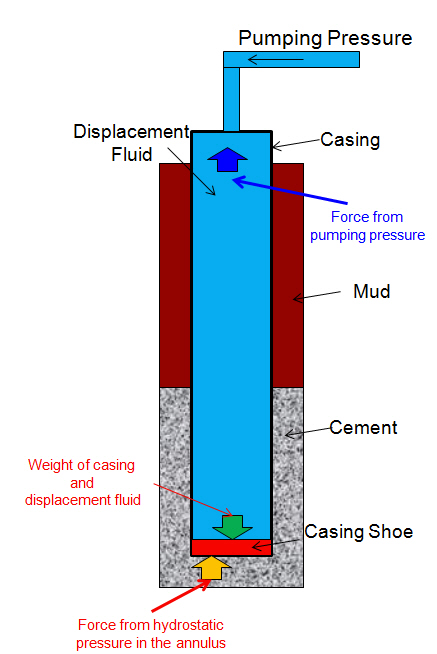# Hydraulicing Casing (pressure to lift the casing while cementing)

During cementing operation, pumping cement and displacement fluid can lift the casing. Some people call “pump out of the well”. This situation can be occurred and there are some reasons as follows:

• Lightweight casing string

• Large casing diameter

• High density of cement

• Low density of displacement fluid

• High annular pressure loss

• High pressure trapped in the annulus due to bridging

### How is the casing lifted during cementing?

Under A Static Condition

The casing can be hydraulic out of hole due to unbalance of hydrostatic pressure. See the figure below for more understanding.You can write in the mathematical equation like this.

∆F = (Wc+Wdf) – (HPann x A)

Where:

Wc = Weight of casing

Wdf = Weight of displacement fluid

HPann = Hydrostatic Pressure in the annulus

A = Cross section area of casing

Note: the equation neglects effect of frictional force and buoyancy effect.

If you get negative value, your casing will be lifted out of the hole.

Under A Dynamic Condition

While pumping, you need to consider pumping pressure because hydraulic pressure while pumping also forces against the cross section area of casing.∆F = (Wc+Wdf) – (HPann x A + Ppump x a)

Where:

Wc = Weight of casing

Wdf = Weight of displacement fluid

HPann = Hydrostatic Pressure in the annulus

a = Cross section area of inside of casing

Ppump = pumping pressure

A = cross sectional area of casing

Note: the equation neglects effect of frictional force and buoyancy effect.

Ref books:Cementing Technology Books

Share the joy